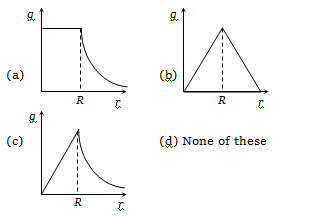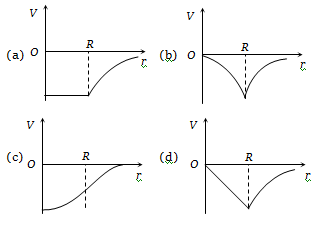In an elliptical orbit under gravitational force, in general

(a) Tangential velocity is constant

(b) Angular velocity is constant

(d) Areal velocity is constant

Concept Questions :-

Kepler laws

Difficulty Level:

Earth is revolving around the sun, if the distance of the Earth from the Sun is reduced to 1/4th of the present distance then the present year length reduced to -

(a)$\frac{1}{4}$                                         (b)$\frac{1}{2}$

(c)$\frac{1}{8}$                                         (d)$\frac{1}{6}$

Concept Questions :-

Kepler laws
High Yielding Test Series + Question Bank - NEET 2020

Difficulty Level:

Two satellite are revolving around the earth with velocities ${v}_{1}$ and ${v}_{2}$ and in radii ${r}_{1}$ and ${r}_{2}\left({r}_{1}>{r}_{2}\right)$respectively. Then -

(a)${v}_{1}={v}_{2}$                                 (b)${v}_{1}>{v}_{2}$

(c)${v}_{1}<{v}_{2}$                                 (d)$\frac{{v}_{1}}{{r}_{1}}=\frac{{v}_{2}}{{r}_{2}}$

Concept Questions :-

Orbital velocity
High Yielding Test Series + Question Bank - NEET 2020

Difficulty Level:

If orbital velocity of planet is given by $v={G}^{a}{M}^{b}{R}^{c}$, then -

(a)

(b)

(c)

(d)

Concept Questions :-

Orbital velocity
High Yielding Test Series + Question Bank - NEET 2020

Difficulty Level:

The mass of a planet that has a moon whose time period and orbital radius are T and R respectively can be written as -

(a)$4{\mathrm{\pi }}^{2}{\mathrm{R}}^{3}{\mathrm{G}}^{‐1}{\mathrm{T}}^{‐2}$                                 (b)$8{\mathrm{\pi }}^{2}{\mathrm{R}}^{3}{\mathrm{G}}^{‐1}{\mathrm{T}}^{‐2}$

(c)$12{\mathrm{\pi }}^{2}{\mathrm{R}}^{3}{\mathrm{G}}^{‐1}{\mathrm{T}}^{‐2}$                               (d)$16{\mathrm{\pi }}^{2}{\mathrm{R}}^{3}{\mathrm{G}}^{‐1}{\mathrm{T}}^{‐2}$

Concept Questions :-

Satellite
High Yielding Test Series + Question Bank - NEET 2020

Difficulty Level:

The eccentricity of earth's orbit is 0.0167. The ratio of its maximum speed in its orbit to its minimum speed is

(a)   2.507                                             (b)   1.033

(c)   8.324                                             (d)   1.000

Concept Questions :-

Kepler laws
High Yielding Test Series + Question Bank - NEET 2020

Difficulty Level:

Assuming the earth to have a constant density, point out which of the following curves show the variation of acceleration due to gravity from the centre of earth to the points far away from the surface of earth -Concept Questions :-

Acceleration due to gravity
High Yielding Test Series + Question Bank - NEET 2020

Difficulty Level:

The radius of orbit of a planet is two times that of the earth. The time period of planet is -

(a)   4.2 years                           (b)   2.8 years

(c)   5.6 years                           (d)   8.4 years

Concept Questions :-

Kepler laws
High Yielding Test Series + Question Bank - NEET 2020

Difficulty Level:

The diagram showing the variation of gravitational potential of earth with distance r from the centre of earth is -Concept Questions :-

Gravitational Potential
High Yielding Test Series + Question Bank - NEET 2020

Difficulty Level:

The orbital angular momentum of a satellite revolving at a distance r from the centre is L. If the distance is increased to 16r, then the new angular momentum will be -

(a)   16 L                                   (b)   64 L

(c)    $\frac{L}{4}$                                     (d)   4 L

Concept Questions :-

Orbital velocity